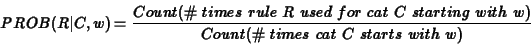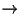Next: Summary Up: Chapter7Ambiguity Resoltion: Statistical Methods Previous: BOX 7.2 Handling Unkown

# 7.7 A Simple Context-Dependent Best-First Parser

• best-first algorithm $B$O(B $B8zN($h$$(B parse BNeKDJ,k(B algorithm BGOL5$$(B
• context-dependent $B$J(B lexical information $B$K4X$9$k3NN($r;H$C$C$F(B $B@:EY8~>e$r;n$_$k(B
• Category C $B$,(B $BC18l(B w $B$G;O$^$k(B rule Ri $B$GE83+$5$l$?3NN((B $B$r5a$a$k(BFigure 7.23
• house vs. peaches
house $B$O(B NPN N $B$K$J$j$d$9$$(B (0.82) peachesBO(B NPN BKJjd9$$(B (0.65)
• like vs. put
like $B$O(B VPNP $B$K$J$j$d$9$$(B (0.9) put BO(B VPV NP PP BKJjd9$$(B (0.93) (put $B!A(B in) • context-dependent $B$J3NN($r;H$&$H@:EY$,8~>e$9$k(B 49%66% $B@:EY$N8~>e$NB>$K8zN(E*$J2r@O$b9T$&$3$H$,$G$-$k(B, chart $B?t(B 6536 (Figure 7.24) • $B$J$<@:EY$,8~>e$9$k$N$+(B? • The man put the bird in the house. • The man likes bird in the house. $B$rHf$Y$F$_$k(B Figure 7.25, 7.26 • context-free $B$J3NN(CM$rMQ$$kH(B, B3FC18lr8+J$$$N$G(B,2$B$D$H$b(B $BF1$8(B parse tree $B$,F@$i$l$k(B$B$^$A$,$$(B • Bcontext Br9MN87?[&,$$$$K-^CF$$$k(B
• $B$5$i$K8-$/$9$k$?$a$K$O(B? • w $B$N$_$r8+$F$$?(B (unigram) B3NN(N7W;;r(B bigram Bd(B trigram BK3HD%9k(B (B?@7(B BB?NLN(B traing data B,I,MW(B) • VPV NP PP BH$$$&$h$&$J(B rule $B$N>l9g(B V $B$@$1$G$J$/(B PP $B$N ($BLdBjE@(B: VPV NP $B$H$N3NN($NHf3S$,:$Fq$K$J$k(B)
• closed class words
prepositions, articles, quantifiers, conjunctions$B$3$l$i$O(B enumerate $B$G$-$k$N$G4JC1$K?dDj2DG=(B
• open class words
$B$[$s$H$O$3$l$i$b8DJL$K07$$?$$$N$@$1$I(B, data sparseness $B$N(B $BLdBj$GFq$7$$(B • BHs>oKh/=PF/kC18lN_r8DJLK07&(B • B (BF0:nr<(9F0;lrRHDN(B class BK^HaF3liOF18?6k(B BIq$$$r$9$k\$H2>Dj(B ..etc)

1999-08-03